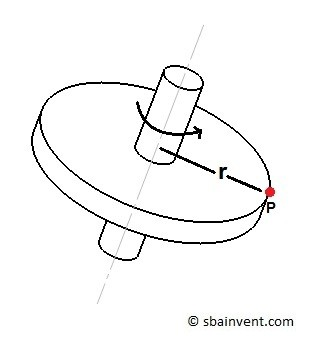# Kinematics of a Rigid Body

In previous articles I focused mainly on how to calculate the motion of a particle.  Now you will learn how to solve problems that have bodies that consist of many particles  For this type of problem you will have to use kinematics of a rigid body instead of kinematic of a particle.

First, let’s talk about why you will need to know how to solve rigid body problems.  As a mechanical engineer, you will come across real world designs like mechanism, gearing systems, and possibly cams.  All of these designs can have complex motion.  If you want to find the motion of a specific point in these systems you will need to know how to use kinematics of a rigid body. This will allow you to determine position, velocity, and acceleration.  After you determine the acceleration you can than use kinetics to determine forces.

There are three areas of kinematics of a rigid body that I will be focusing on in later sections.  They will be the following.

###### Translation

A rigid body will be in translation when the paths of all of the particles in the body are parallel with each other.  If you observe that two particle are moving along equidistant straight lines than the rigid body has rectilinear translation.  While on the other hand if you observe two particles that are moving along curved lines that are equidistant with each other than the rigid body has curvilinear translation.The image above is an example of translation.  You can see that the paths that points A and B are following are parallel to each other other as the body moves.

###### Rotation about a Fixed Axis

Another type of motion for a rigid body would be rotation about a fixed axis.  A rigid body that is rotating about a fixed axis will have all of the particles, except those on the axis, moving along a circular path.The image above is an example or rotation about a fixed axis.  You can see that particle P is moving along a circular path.  However, if you were to select a particle that is on the axis there will be no motion.

###### General Plane Motion

Finally, general plane motion isn’t a unique form of motion.  Instead general plane motion will be a combination of translation and rotation about a fixed axis.  General plane motion is what you will observe for most real life problems.

Next

|